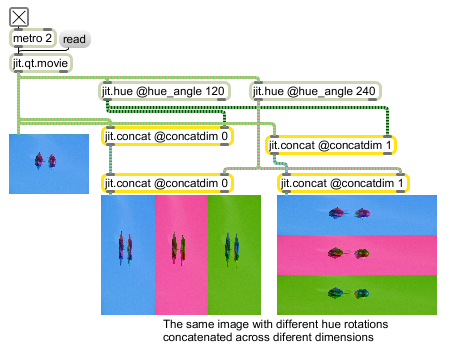jit.concat

Concatenate two matrices

Description

The jit.concat object takes two matrices as input, and sends a single concatenated matrix out.

Matrix Operator

matrix inputs:2, matrix outputs:1
 Name IOProc Planelink Typelink Dimlink Plane Dim Type in2 adapt 0 0 0 1 1 char long float32 float64 out n/a 0 0 0 1 1 char long float32 float64

Attributes

Name Type g/s Description
autoclear int Matrix clear following output flag (default = 1)
concatdim int The dimension across which concatenation occurs (default = 0)
mode int Concatenation order flag (default = 0)
0 = append the matrix entering the right inlet to the matrix entering the left inlet
1 = append the matrix entering the left inlet to the matrix entering the right inlet
truncate int Matrix truncation flag (default = 1) When the flag is set, the right input's dimensions are truncated to the left input's dimensions if the right dimensions are greater than the left for all dimensions except the concatenation dimension.

Examples